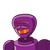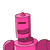# the sum of a natural number and its reciprocal is 10/3.find the number​

the sum of a natural number and its reciprocal is 10/3.find the number​

### 2 thoughts on “the sum of a natural number and its reciprocal is 10/3.find the number​”

1.the natural number is 3

Step-by-step explanation:

3+1/3=9+1/3

=10/3

2.